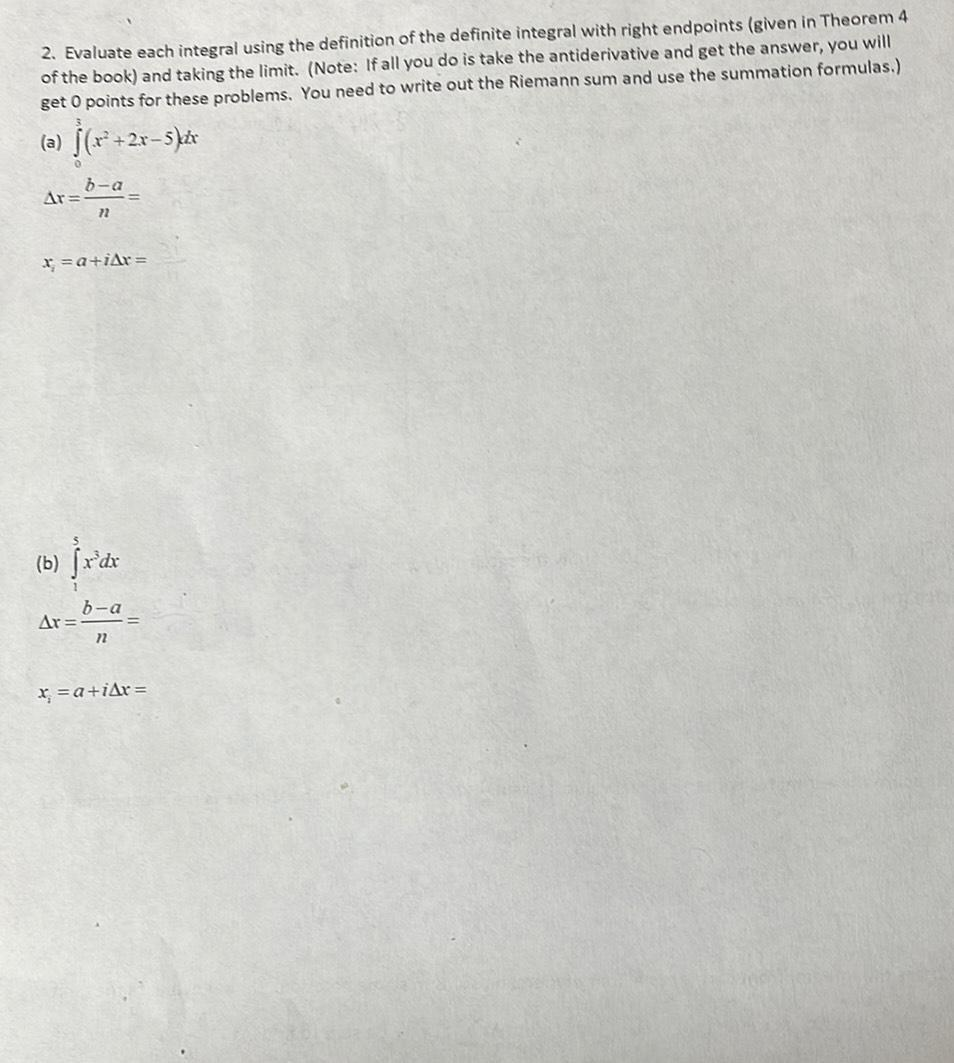Question:

# Evaluate each integral using the definition of the definite integral with right endpoints (given in Theorem 4 of the book) and taking the limit. (Note: If all you do is take the antiderivative and getEvaluate each integral using the definition of the definite integral with right endpoints (given in Theorem 4 of the book) and taking the limit. (Note: If all you do is take the antiderivative and get the answer, you will get 0 points for these problems. You need to write out the Riemann sum and use the summation formulas.) (a) ³∫₀(x² +2x–5]dx △x=(b-a)/n= xᵢ=a + i△x= (b) ₁∫⁵ x³dx △x=(b-a)/n= xᵢ=a + i△x=